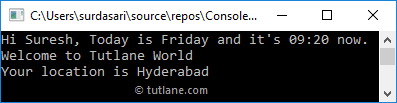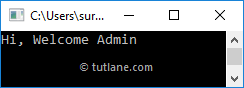# Visual Basic String Interpolation (\$)

In visual basic, String Interpolation is a feature which is useful to embed any valid expression that returns a value into a string using `\$` operator. The string which is prepended with `\$` operator will call it as an interpolated string and this feature is available from the latest versions.

In visual basic, the String Interpolation is an extended version of the String.Format() feature to provide a more readable and convenient way to include formatted string expression results into a string literal.

For example, by using String.Format() method we can insert the values in a string like {0}, {1}, etc. but by using String Interpolation we can directly include any valid visual basic expression that returns a value in an interpolated string using braces like {name} or {expression}.

Following is the example of using both String.Format() and String interpolation in visual basic to include expression values inside of a string.

Dim name As String = "Suresh"

Dim date1 As DateTime = DateTime.Now

' Normal formatting

Dim strFormat As String = String.Format("Hi {0}, Today is {1} and it's {2:HH:mm} now.", name, Date.Now.DayOfWeek, Date.Now)

Console.WriteLine(strFormat)

' Output: Hi Suresh, Today is Friday and it's 05:38 now.

' String interpolation

Dim strIntr As String = \$"Hi {name}, Today is {Date.Now.DayOfWeek} and it's {Date.Now:HH:mm} now."

Console.WriteLine(strIntr)

' Output: Hi Suresh, Today is Friday and it's 05:38 now.

If you observe the above examples, we used a string.Format() method with {0}, {1}, {2} to embed values in string but with String Interpolation we directly included a valid visual basic expressions within the string.

## Visual Basic String Interpolation Structure

In visual basic, to identify a string literal as an interpolated string, we need to prepend it with `\$` symbol and there should not have any whitespace between the `\$` symbol and the double quote `"`, that starts a string literal.

Following is the structure of interpolated string with required expressions in a visual basic programming language.

{<interpolatedExpression>[,<alignment>][:<formatString>]}

Here the elements in square brackets `[]` are optional. The alignment and formatString elements are useful to format and adjust the alignment of the interpolated string based on our requirements.

The following table lists the details about each element interpolated strings in c#.

ElementDescription
interpolatedExpression The expression that produces a result and it will be included in an interpolated string with braces {}
alignment It's a constant expression and it is used to specify an alignment for expression result using comma(,). If the alignment value is positive, then the formatted expression result is right-aligned; if negative, then it's left-aligned.
formatString A format string that is supported by the type of expression result. To format given expression, we can define formatString with interpolated expression using a colon (":").

Following is the example of using optional formatting components in the interpolated string.

Dim x As Double = 12.34562

Dim y As Double = 6.032592

Console.WriteLine(\$"{x} + {y} = {x + y}")

Console.WriteLine(\$"Sum with Format = {x + y,10:F2}")

' Output:

' 12.34562 + 6.032592 = 18.378212

' Sum with Format =      18.38

If you observe the above example, we used an optional formatting components to format the expression. By using alignment element (10), we made expression result is right-aligned and by using format string (F2) we limited an expression result to 2 decimal points.

## Visual Basic String Interpolation Example

Following is the example of creating string interpolation strings in visual basic.

Module Module1

Sub Main(ByVal args As String())

Dim name As String = "Suresh"

Dim date1 As DateTime = DateTime.Now

Dim x As Double = 12.34562

Dim y As Double = 6.032592

Console.WriteLine(\$"Sum of {x} + {y} = {x + y}")

Console.WriteLine(\$"Right Align with Format = {x + y,10:F2}")

Console.WriteLine(\$"Left Align with Format = {x + y,-10:F4}")

Dim strIntr As String = \$"Hi {name}, Today is {Date.Now.DayOfWeek} and it's {Date.Now:HH:mm} now."

Console.WriteLine(strIntr)

End Sub

End Module

If you observe the above example, we defined the interpolated strings using dollar (`\$`) character, specified alignment, and format based on our requirements.

When we execute the above visual basic program we will get the result like as shown below.

Sum of 12.34562 + 6.032592 = 18.378212

Right Align with Format =      18.38

Left Align with Format = 18.3782

Hi Suresh, Today is Saturday and it's 12:07 now.

If you observe the above result, the interpolated expression results are aligned right or left based on the alignment value and decimal points also limited based on the defined format.

## Visual Basic Multiline String Interpolation

In visual basic, the String Interpolation will support multiline interpolated string. Following is the example of implementing a multiline or verbatim interpolated string in visual basic.

Module Module1

Sub Main(ByVal args As String())

Dim name As String = "Suresh"

Dim location As String = "Hyderabad"

Dim date1 As DateTime = DateTime.Now

Dim msg As String = \$"Hi {name}, Today is {Date.Now.DayOfWeek} and it's {Date.Now:HH:mm} now.

Welcome to Tutlane World

Console.WriteLine(msg)

End Sub

End Module

If you observe the above example, we implemented a multiline interpolated string using `\$` character.

When we execute the above visual basic program, we will get the result like as shown below.This is how we can implement a multiline interpolated string in a visual basic programming language based on our requirements.

## Visual Basic String Interpolation with Ternary Operator (?:)

In visual basic, we can use conditional ternary operator in interpolated expressions by enclosing it in parenthesis `{}`.

Following is the example of using conditional ternary operator in visual basic string interpolated expression.

Module Module1

Sub Main(ByVal args As String())

Dim name As String = "Suresh"

Dim msg As String = \$"Hi, Welcome {(If(name = "Suresh", "Admin", "Guest"))}"

Console.WriteLine(msg)

End Sub

End Module

If you observe the above example, we defined a conditional ternary operator in interpolated expression with parenthesis `{}`.

When we execute the above visual basic program we will get the result like as shown below.This is how we can use conditional ternary operator in string interpolation based on our requirements.

## Visual Basic String Interpolation Performance

In visual basic, the string interpolation performance is almost same as String.Format() method. There is a tiny bit of overhead for the string interpolation in visual basic due to expressions embedding in the string but that is very, very small.

In performance point of view, both string interpolation and String.Format() are not suggestible instead, you can use StringBuilder. This is how we can use string interpolation in visual basic to include valid expressions within the string based on our requirements.